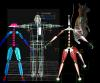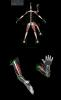## AS3 Flash Target Seeking Arrow Code

//copy paste
stage.frameRate = 60;
var points:Number = stage.stageWidth/6;
var pointsVector:Vector.<Point> = new Vector.<Point>();
for(var s:Number = 0; s<stage.stageWidth/points+points; s++){
var originProjectile:Point = new Point(points*s,stage.stageHeight);
pointsVector.push(originProjectile);
}
var targetSpeedX:Number = 5;
var targetSpeedY:Number = 5;
var seekX:Number = 0;
var seekY:Number = 0;
var projectileVector:Vector.<Sprite> = new Vector.<Sprite>();

## AS3 Shooting Multiple Arrows to a Target Code

//copy paste
//change MOUSE_DOWN to MOUSE_MOVE again some lovely effects: http://www.shacarah.com/portfolio/?q=node/139

stage.frameRate = 60;
var gravity:Number = 9.8;
var time:Number = 0;
var pointsVector:Vector.<Point> = new Vector.<Point>();
var originProjectile:Point = new Point(stage.stageWidth/2,stage.stageHeight);
pointsVector.push(originProjectile);
var originProjectile2:Point = new Point(0,stage.stageHeight);
pointsVector.push(originProjectile2);

## AS3 Flash Parabola Arrow Code/Tutorial

Click stage

//copy paste
//move an arrow along the path of parabola (just the idea of moving an object along a parabola hitting the vertex exactly)
//if this is confusing read this one first: http://www.shacarah.com/portfolio/?q=node/136
//change stage event to MOUSE_MOVE to get the pretty parabola effect: http://www.shacarah.com/portfolio/?q=node/137
//other arrow fun: http://www.shacarah.com/portfolio/?q=node/139
stage.frameRate = 60;
var h:Number;
var k:Number;
var a:Number;
var Y:Number = stage.stageHeight;

## AS3 Flash Drawing Parabola Code/Tutorial

//animate arrow along a parabola: http://www.shacarah.com/portfolio/?q=node/135

//plot parabola points, draw parabola, animate ball using the formula
//copy paste
stage.frameRate = 60;
//draw a parabola
//because here we are dealing with coordinates from (0 to stage width, 0 to stage height) starting from the upper left hand corner lets use the equation below where we can specify the vertex using h and k
//parabola equation, vertex form: Y = a(X – h)^2 + k
//(h,k) is the vertex
//h is the center of the parabola on x
var h:Number = stage.stageWidth/2;

## AS3 Flash Rain/Dripping Code/Tutorial (or basic falling whatever you want code)

//splashing rain code can be found here: http://www.shacarah.com/portfolio/?q=node/118
//copy and paste
//look up any objects density (water has a density of 1) and drag coefficient (http://en.wikipedia.org/wiki/Drag_coefficient)
//changing the stage dimensions will not affect the code
// time is derived from 60 frames per second in the calculations
stage.frameRate = 60;
// holds the falling objects
var dropsVector:Vector.<Sprite> = new Vector.<Sprite>();
// holds the time for each object - the calculations on enter frame below are all functions of time

## AS3 Flash More randomness motion slingshot

Messing around, all api drawing - curveTo based on ball clip location

## AS3 Flash Parabola Drawing

If your looking for simple parabola drawing code: http://www.shacarah.com/portfolio/?q=node/136
Animate arrow along a parabola: http://www.shacarah.com/portfolio/?q=node/135

While this is strange - uh it was an experiment in parabola drawing - a 360 degree rotating parabola drawn at run-time based on the circular clip location - added a little cosine function to get the weird spring back.

Part of the code: http://www.shacarah.com/portfolio/?q=node/127

## Rigging• 3d rigs created in 2006 - 2008, from left:
• 3ds Max rig, created from standard bones
• custom controllers
• Maya game character rig, set up according to engine specifications
• 3ds Max complex muscle rig
• custom controllers, ie hand splay, fist etc
• Maya squirrel rig
• complex rig to set up as the squirrel needs to move both as a quadruped and biped

## Muscle Rig• 3ds Max muscle rig 2006
• sample image demonstrates how the mesh conforms to muscle flexes in the muscle rig creating a more realistic animation
• rig has many custom controllers the hand controls are the most interesting; fist, splay, etc